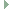OGRE  13.4 Object-Oriented Graphics Rendering Engine
Ogre::WaveformControllerFunction Class Reference

Predefined controller function based on a waveform. More...

#include <OgrePredefinedControllers.h>Inheritance diagram for Ogre::WaveformControllerFunction:

## Public Member Functions

WaveformControllerFunction (WaveformType wType, Real base=0, Real frequency=1, Real phase=0, Real amplitude=1, bool deltaInput=true, Real dutyCycle=0.5)

Real calculate (Real source)Public Member Functions inherited from Ogre::ControllerFunction< Real >
ControllerFunction (bool deltaInput)
Constructor. More...

virtual ~ControllerFunction ()

## Static Public Member Functions

static ControllerFunctionRealPtr create (WaveformType wType, Real base=0, Real frequency=1, Real phase=0, Real amplitude=1, bool deltaInput=true, Real dutyCycle=0.5)
Default constructor, requires at least a wave type, other parameters can be defaulted unless required. More...

## Detailed Description

Predefined controller function based on a waveform.

A waveform function translates parametric input to parametric output based on a wave.

Note that for simplicity of integration with the rest of the controller insfrastructure, the output of the wave is parametric i.e. 0..1, rather than the typical wave output of [-1,1]. To compensate for this, the traditional output of the wave is scaled by the following function before output:
output = (waveoutput + 1) * 0.5
Hence a wave output of -1 becomes 0, a wave output of 1 becomes 1, and a wave output of 0 becomes 0.5.

## ◆ WaveformControllerFunction()

 Ogre::WaveformControllerFunction::WaveformControllerFunction ( WaveformType wType, Real base = 0, Real frequency = 1, Real phase = 0, Real amplitude = 1, bool deltaInput = true, Real dutyCycle = 0.5 )

## ◆ create()

 static ControllerFunctionRealPtr Ogre::WaveformControllerFunction::create ( WaveformType wType, Real base = 0, Real frequency = 1, Real phase = 0, Real amplitude = 1, bool deltaInput = true, Real dutyCycle = 0.5 )
inlinestatic

Default constructor, requires at least a wave type, other parameters can be defaulted unless required.

Parameters
 wType the shape of the wave base the base value of the output from the wave frequency the speed of the wave in cycles per second phase the offset of the start of the wave, e.g. 0.5 to start half-way through the wave amplitude scales the output so that instead of lying within [0,1] it lies within [0,1] * amplitude deltaInput If true, signifies that the input will be a delta value such that the function should add it to an internal counter before calculating the output. dutyCycle Used in PWM mode to specify the pulse width.

## ◆ calculate()

 Real Ogre::WaveformControllerFunction::calculate ( Real source )
virtual

The documentation for this class was generated from the following file: